# Sinusoidal Frequency Estimation Based on Time-Domain Samples

The topic of estimating a noise-free real or complex sinusoid's frequency, based on fast Fourier transform (FFT) samples, has been presented in recent blogs here on dsprelated.com. For completeness, it's worth knowing that simple frequency estimation algorithms exist that do not require FFTs to be performed . Below I present three frequency estimation algorithms that use time-domain samples, and illustrate a very important principle regarding so called "exact" mathematically-derived DSP algorithms.

Here, as shown in Figure 1, we assume a time-domain real sinusoidal input sequence is represented by: $$x(n) = Asin(2{\pi}nf/f_s + \phi)$$ where n is the integer time-domain index and the sinusoid's frequency f and the sample rate fs are measured in Hz.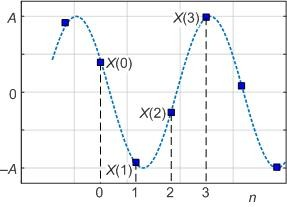Figure 1: Consecutive periodically-spaced x(n) samples
of a real-valued sinusoidal sequence.

OK, let's consider three time-domain frequency estimation algorithms that estimate sinusoidal signal frequency based on the x(n) samples in Figure 1.

Real 3-Sample Frequency Estimation

DSP guru Clay Turner provides a method for estimating a real-valued sinusoid's frequency based on three time domain samples . Turner's Real 3-Sample algorithm is:

Real 3-Sample:   $f = \frac{f_s}{2\pi} cos^{-1}(\frac{x(0)+x(2)}{2x(1)}) \tag{1}$

After experimenting with Eq. (1) I learned it can give correct results assuming the 3-Sample x(n) input sequence satisfies the following restrictions:

* x(n) samples are real-valued and noise free,
* Nyquist sampling criterion is satisfied,
* Peak amplitude of x(n) is constant,
* DC component of x(n) is zero,
* Sample x(1) is not equal to zero.

Real 4-Sample Frequency Estimation

Turner also provides a method for estimating a real-valued sinusoid's frequency based on four time domain samples . Turner's Real 4-Sample algorithm is:

Real 4-Sample: $f = \frac{f_s}{2\pi} \cdot cos^{-1}[\frac{1}{2}(\frac{x(3)-x(0)}{x(2)-x(1)}-1)] \tag{2}$

Equation (2) can provide correct results assuming the 4-Sample x(n) input sequence satisfies the following restrictions:

* x(n) samples are real-valued and noise free,
* Nyquist sampling criterion is satisfied,
* Peak amplitude of x(n) is constant,
* x(1) ≠ x(2).

Unlike Eq. (1), the Eq. (2) method can be applied when the x(n) sequence rides on a DC bias.

Complex 2-Sample Frequency Estimation

DSP wizard Dirk Bell recently showed me his two-sample method for estimating the frequency of a complex-valued noise-free sinusoid (reminiscent of complex-valued FM demodulation methods). Bell's algorithm to compute frequency f is:

$$f = (\frac{f_s}{2\pi}) \cdot tan^{-1}[imag(x(n) \cdot x(n-1)^*)/real(x(n) \cdot x(n-1)^*)] \tag{3}$$

where the '*' symbol means conjugate. The derivation of Eq. (3) is given in Appendix A. Equation (3) can provide correct results assuming the complex $x(n)$ input sequence satisfies the following restrictions:

* $x(n) = Me^{j2\pi nf/f_s}$ and noise free,
* Nyquist sampling criterion is satisfied.

An Important Note

Notice that the mathematical derivations of our three algorithms could be called "exact." And by exact I mean no mathematical approximations, such as $sin(x) = x$ for small $x$, were used in their derivations.

Algorithm Performance

The three time-domain frequency estimation algorithms work well when the input sinusoid is noise free. However, sadly, the Real 3-Sample and Real 4-Sample algorithms do not work well at all if the real-valued input sinusoid is contaminated with noise. (Clay Turner never claimed otherwise.) Figure 2 shows the performance of our three algorithms, for 500 individual frequency estimations, when the 700 Hz input sinusoids have a relatively high signal to noise ratio (SNR) of 60 dB.

My "noise" is standard garden variety, wideband, Gaussian-distributed, zero-mean, random noise samples. (The noise sequences used for the real and imaginary parts of the Complex 2-Sample algorithm input signal are independent of each other.) And my definition of input signal SNR is the traditional: $SNR = 10log_{10}(signal variance/noise variance)$.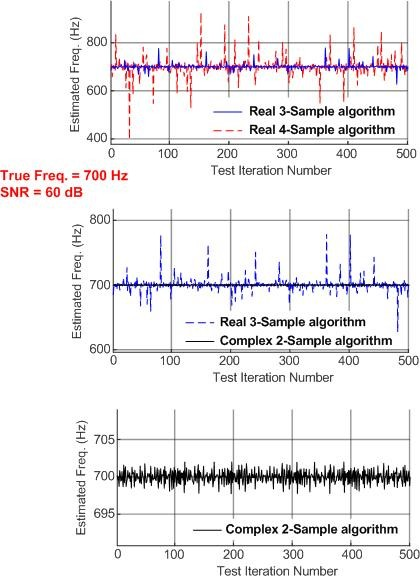Figure 2: Algorithms' frequency estimation performances when the
input sinusoid's frequency is 700 Hz (fs = 8000 Hz)
with an SNR of 60 dB.

Look carefully at the vertical axes' values when comparing the Figure 2 frequency estimation results. In Figure 2 we see the Real 3-Sample and Real 4-Sample algorithms are very poor at frequency estimation when the input sinusoid is contaminated with noise. (The above Figure 2 performance measurements were made with both 16-bit and floating-point numbers. Those two number formats had essentially the same poor performance.)

Figure 2 also shows us the Complex 2-Sample algorithm produces moderately accurate results when the input sinusoid's SNR is 60 dB. Appendix B presents the three algorithms' performances when the input sinusoid's SNR is less than 60 dB.

Software Modeling

If you decide to model the performance of the above Eq. (1) and Eq. (2) algorithms with noise-contaminated real sinusoids, be aware that those methods can produce invalid complex-valued frequency estimates that should be ignored. That happens when the arguments of the $cos^{-1}()$ functions are, due to noisy $x(n)$ samples, greater than unity. You'll often see this situation when the input sinusoids are low-SNR or low-frequency (relative to the Fs sample rate).

Conclusions

I've presented three sinusoidal frequency estimation algorithms, Eqs. (1), (2), and (3), that use time-domain samples as opposed to FFT frequency-domain samples. Those algorithms only provide correct frequency estimation results for ideal noise-free input sinusoids. As such, those algorithms are mostly of academic interest only. (No one claimed those algorithms were of significant practical value.) My thoughts on the problems encountered when using the three algorithms in practical DSP applications are given in Appendix C.

One point I want to make here is this: Although the Real 3-Sample and Real 4-Sample frequency estimation algorithms are mathematically exact, their performance is very poor in the presence of noise. Thus it is risky to assume that mathematically exact DSP algorithms will always be useful in real-world practical signal processing applications .

April 30, 2017 Update: I've continued to learn more about the above Eq. (3). As it turns out, that algorithm is described in the literature of "frequency estimation" and it goes by the name of the "Lank-Reed-Pollon algorithm." In my initial software modeling of Eq. (3) it appeared to me that it provided an unbiased estimate of the frequency of a complex-valued sinusoid. After more thorough modeling I've learned that Eq. (3) is indeed a biased estimator when the input complex-valued sinusoid has a low SNR and is either low in frequency (near zero Hz) or high in frequency (near Fs/2 Hz). This is important because it means that averaging multiple frequency estimation values does not necessarily improve our final frequency estimate's accuracy

Acknowledgment

I thank Dirk Bell for his very useful suggestions regarding the first draft of this blog. I also thank Cedron Dawg for correcting a notational error in the original version of Appendix A.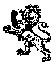References

 Clay Turner, http://www.claysturner.com/dsp/3pointfrequency.pdf
 Clay Turner, http://www.claysturner.com/dsp/4pointfrequency.pdf

Appendix A: Derivation of Eq. (3)

Dirk Bell's derivation of his Eq. (3) expression proceeds as follows: Assume the complex-valued sinusoid of frequency $f$ is described by:

$$x(n) = Me^{j2\pi nf/f_s}.$$

We can, where the '*' symbol means conjugation, write:

$$P = x(n) \cdot x(n-1)^* = Me^{j2\pi nf/f_s} \cdot Me^{-j2\pi (n-1)f/f_s}$$ $$= M^2 \cdot e^{j2\pi [n-(n-1)]f/f_s} = M^2 \cdot e^{j2\pi f/f_s}$$

The radian angle of product P is:

$$arg(P) = 2\pi f/f_s$$ $$= tan^{-1}[imag(x(n) \cdot x(n-1)^*)/real(x(n) \cdot x(n-1)^*)].$$

Arbitrarily assuming index $n = 1$, our desired expression for $f$ is:

$$f = (\frac{f_s}{2\pi}) \cdot tan^{-1}[imag(P)/real(P)]$$ $$= (\frac{f_s}{2\pi}) \cdot tan^{-1}[imag(x(n) \cdot x(n-1)^*)/real(x(n) \cdot x(n-1)^*)].$$

Appendix B: Algorithms' Performances With Low SNR Input Signals

Figure B-1 shows the performance of the algorithms, for 500 individual frequency estimations, when the 700 Hz input sinusoids have an SNR of 30 dB. In this scenario the three algorithms have very poor performance.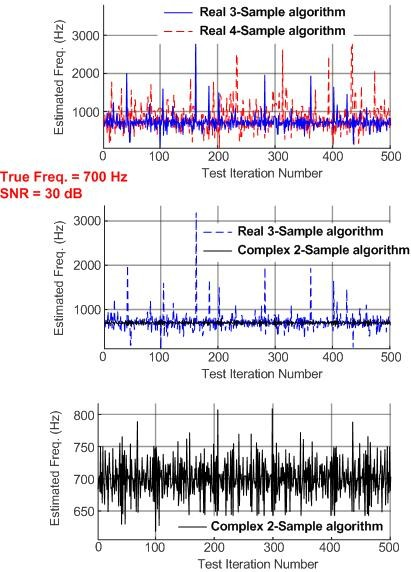Figure B-1: Algorithms' frequency estimation performances when the
input sinusoid's frequency is 700 Hz (fs = 8000 Hz)
with a signal SNR of 30 dB.

Figure B-2 shows the performance of the Real 3-Sample and Complex 2-Sample algorithms, for 500 individual frequency estimations, when the 700 Hz input sinusoids have an SNR of 10 dB. (For reference, the top panel of Figure B-2 shows the difference between a noise-free 700 Hz sinusoid and an SNR = 10 dB sinusoid.) In this scenario the two algorithms have terribly poor performance.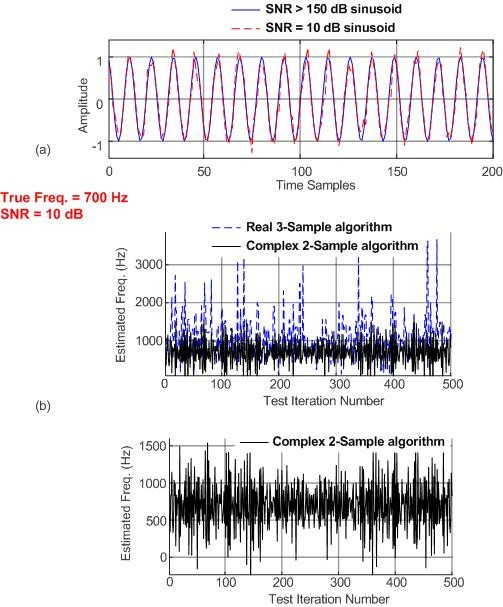Figure B-2: Algorithm performance: (a) comparison of a noise-free
and a noisy input sinusoid; (b) algorithms' frequency
estimation performances when the input sinusoid's
frequency is 700 Hz (fs = 8000 Hz) with a signal SNR of 10 dB.

Appendix C: Practical Problems of the Three Frequency Estimation Algorithms

According to my software modeling, in the presence of noise the Real 3-Sample and Real 4-Sample algorithms typically produce biased results (i.e., the mean of multiple frequency estimations is usually greater then the true input signal's frequency). Thus averaging the results of multiple frequency estimations is not guaranteed to improve their performances. An input signal SNR of roughly 40 dB combined with multiple results averaging is necessary for those algorithms to be just marginally useful. There's more bad news. When the input signal's frequency is less than fs/20 Hz the Real 3-Sample and Real 4-Sample algorithms provide wildly incorrect results.

Unlike the Real 3-Sample algorithm, the Real 4-Sample's performance is not degraded by a DC bias on the input sinusoid but it requires a much higher input signal SNR to achieve comparable performance with the Real 3-Sample algorithm.

The Complex 2-Sample algorithm provides unbiased frequency estimation results, even at low input signal frequencies. So averaging multiple Complex 2-Sample output samples will improve this algorithm's performance. While its performance is superior to the Real 3-Sample and Real 4-Sample algorithms, the disadvantage of the Complex 2-Sample algorithm is that it requires a complex-valued input. So real-valued input sequences must be converted to an analytic complex-valued sequence before the frequency estimation process can begin.

To be truly useful when their input sinusoids are contaminated by noise and narrowband interfering spectral components, our three frequency estimation methods need to be preceded by some sort of SNR-enhancing narrow bandpass filtering.

[ - ]
Comment by April 20, 2017I can't recall exactly where I saw it, but didn't you have a "DSP Tips and Tricks" where you used the time-derivatives of the real and imaginary parts of a complex signal to estimate frequency?  I'd be interested to see how that compares with the data from these methods.
[ - ]
Comment by April 21, 2017Hello dszabo. You may be right, I don't recall.  I'm away from my desktop computer for the next few days so I can't check the old Tips & Tricks articles.  Regarding your question, I'll have to get back to you on that.

[ - ]
Comment by April 27, 2017I actually found it while I was working on something unrelated, but this is the article I was thinking of: http://www.embedded.com/design/configurable-systems/4212086/DSP-Tricks--Frequency-demodulation-algorithms-
[ - ]
Comment by April 27, 2017Hi dszabo. Ah, yes.  I did not write that material for embedded.com. That's an excerpt from my DSP book that my Publisher gave embedded.com permission to reprint without  involving me in the agreement.

[ - ]
Comment by April 21, 2017How about an overlapping, interleaved binned all-pass NB filter bank, using a PLL to shift bin?

[ - ]
Comment by April 24, 2017If you're going to have a PLL in the mix, how about slaving to the incoming signal with the PLL, and reading the frequency off of the command to the NCO?

[ - ]
Comment by April 24, 2017You do realize that the 3-measurement method does not work for $y_1 = 0$, yes?  Mathematically it hits a singularity, which is not relieved by using the arc-secant.  Intuitively, if $y_1 = 0$ then the triple $\left(y_0, y_1, y_2 \right)$ describe a straight line -- without amplitude information, no frequency measurement is possible.

[ - ]
Comment by April 24, 2017Yes, I did realize that.  In my blog I listed the restriction that the 3-Sample algorithm is not usable when sample y1 = 0.  Of course sample y1 = 0 would rarely occur. Much more likely, as I encountered, is the unpleasant numerical situation I described in the Software Modeling section of my blog.

[ - ]
Comment by April 24, 2017Hey Rick:

I didn't see anything in there about the spacing of the samples.  It would seem that samples spaced about $\frac{\pi}{2}$ radians apart would be the most noise-proof, and that samples that are spaced really tightly would result in more noise sensitivity in those portions of the waveform where the sinewave is going through its inflection point and is thus mostly a straight line.

Yes?  No?  Haven't thought about it?

[ - ]
Comment by April 24, 2017In my software modeling I found that the 3-Sample and 4-Sample algorithms had particularly poor performance at low input signal frequencies. In general, at input freqs less than, say, Fs/10 (and input SNR = 30 dB) those two methods were essentially unusable.

[ - ]
Comment by May 2, 2017At low frequencies, you can use these generalizations of (1) and (3).

Real signal:

$$f = \frac{f_s}{2\pi} \cdot \frac{1}{m} \cdot cos^{-1} \left[\frac{x(n)+x(n-2m)}{2x(n-m)}\right]$$

This equation will be most noise resistant when x(n) and x(n-2m) are near zero crossings and x(n-m) is at a peak.   (This corresponds to $\pi/2$ sample spacing.)

Complex signal:

$$f = \frac{f_s}{2\pi} \cdot \frac{1}{m} \cdot arg\left[x(n) \cdot x^*(n-m)\right]$$

Ced

[ - ]
Comment by May 9, 2017Hi.  For an N-length input sequence we can compute N-1 phase shift values and average those N-1 values to achieve some noise reduction.  It seems to me that using an 'm' value greater than one means that we'd be averaging fewer than N-1 phase values and not achieving as much noise reduction by way of averaging. I need to experiment a little with those whole "m > 1" idea.
[ - ]
Comment by May 10, 2017Conceptually, it is the same as having a lower sampling rate.

You can also average three adjacent points (or five or seven if the spacing is tight enough) to make a single value at the center location.  This will reduce the noise significantly.  For the complex case, exactness is not lost with regards to the formula, for the real case you are left with a very accurate approximation when the point is near a zero crossing.

This is equivalent to running a boxcar smoothing filter on the signal first.

The first equation also happens to work for complex signals.  I did not realize that when I wrote the comment.  If the numerator of the inverse cosine argument is zero, then the denominator doesn't really matter as long as it it non-zero.

Thus if you select "m" such that the three points span half a cycle (real or complex case), the center point's noise is either completely or nearly insignificant.  The real case needs to be centered on a peak for the numerator to be near zero.

I would still use the latter formula for complex signals though.

Ced

[ - ]
Comment by June 18, 2017Another important consideration is the arguable existence of the Fourier Transform for sinusoids.   Since sinusoids are neither absolutely integrable nor square integrable, the existence of its Fourier Transform relies on a bit of hand-wavy tricks with "generalized functions" and the Dirac delta.   Obviously it is functionally consistent and useful to do so, but it does mean that the Fourier Transform of a sinusoid is arguably an estimate (both mathematically and practically) rather than anything "exact".   This means that anything "exact" using the FT of a sinusoid is academic.

I think this supports your point.   For engineering and DSP this is not a hindrance at all, since nearly everything is an estimate and estimating parameters to required or useful tolerances is still quite practical.

[ - ]
Comment by July 21, 2017The doubts you cast on FT exactness do not apply to the discrete case.

The importance of exactness as a quality of a formula is not that it is an indication that it is somehow more robust (which is Rick's point in this article), or that it is always the best solution in a given set of circumstances.  Mathematics is not only a way to obtain a numerical results, but it is also a descriptive language.  This is particularly true of differential equations.  When you find an exact solution for a problem you are also finding a true description.  When you find an approximation as a solution, you are only finding a behavioral description, likely within a limited parameter range.

In practical applications, you should know whether the math you are using is exact or an approximation.  If it is the latter, you also need to be aware of the possible error range of the approximation and evaluate that against your tolerances.  In either case, you still have to evaluate your solution for sensitivity to variation of your input values.

Often times, approximations are computationally less intensive, comparable in robustness or even superior, and accurate enough to be the preferred choice.  The distinction between an exact solution and an approximation is not as important in an application as it is in the theoretical understanding of the principles underlying the system being analyzed.

If your goal is to understand something, exactness is important.  If it just to get a job done, not so much.  It's as simple as that.

Ced

P.S.  For those who are interested, my last three blog articles are extensions of some of the material presented in this article.

"Exact Near Instantaneous Frequency Formulas Best at Peaks (Part 1)"
"Exact Near Instantaneous Frequency Formulas Best at Peaks (Part 2)"
"Exact Near Instantaneous Frequency Formulas Best at Zero Crossings"

They can all be found, with the rest of my articles, here:

https://www.dsprelated.com/blogs-1/nf/Cedron_Dawg....
[ - ]
Comment by March 26, 2018Hi. It is real Clay Turner algorithm? I found this algorithm in Vizineanu articles published in Measurement journal? Similar algorithm was published by Seyedi.

[ - ]
Comment by March 26, 2018Hello Serek. I'm willing to wager that DSP Guru Clay Turner derived his algorithms "on his own" and did not plagiarize the work of anyone else.

[ - ]
Comment by March 26, 2018Thank you very much for your response. This algorithm is very important to me because I use them in my research.

[ - ]
Comment by August 21, 2018I had to do frequency estimation recently and was fortunate enough to be dealing with a complex signal, which makes this whole problem relatively trivial.  Well, actually, it was 3 phase power, which you can convert to DQZ, direct+quadrature+common and use the D and Q as complex (the common mode signal, Z, should ideally be zero anyway, but if not, you've separated it out so that D and Q basically do not suffer from any DC offset).

The obvious approach is to take points at some distance apart and consider the difference in their phase angles as giving you an instantaneous angular velocity (for the time at half-way between the samples).  In my case, to minimize quantization error, samples were compared at an offset of the half-period of the highest reasonable frequency, for which the signals were pre-filtered (and you lose a half-period from the length to account for the comparison spread).

Formula 3 is somewhat nice because it means you can call arctan just once instead of twice.  I was sticking with clarity and using the difference of two phase values from arctan.

At least in that approach, a potential source of error will be the 2PI wrap-around of arctan angles.  I wonder if the formula 3 approach magically deals with that problem?  I kind of doubt it.

Consider a primary frequency close to the frequency whose half-period you use as your sample-offset (to compare sample pairs).  Any harmonics or higher-frequency noise will make the angular velocity sometimes larger and sometimes smaller than the nominal value which is already close to +PI or -PI, and thus it can cause it to cross over and change sign.  If you simply average those together, you end up with something close to 0, which is nearly 180 degrees (sorry, PI radians) away from reality!  In my case, I had already low-pass filtered the 3 original signals (before the D,Q transform), and the cut-off was higher than any reasonable expectation for a power frequency, but still, it's a possibility, especially with strong harmonics (commonly at their strongest at 5x and 7x).  It's easy to see how this gives errors when your angular velocity can change sign, but if your spacing is small (like a single-sample offset), I can't picture how that could be a problem.  In my case, it was a possibility.

To deal with that, I decided to average the positive angle differences separately from the negative angle differences, and whichever one had more instances (positive or negative), I would take that average and then push the other average across by 2PI to make it commensurate with the rest, to come up with an adjusted (and properly weighted) average.  Of course, computationally, I just kept the positive and negative sums along with the positive and negative counts, which makes it all quite trivial.  Then that adjusted average was to serve as an overall estimate or "target" angular velocity.

Then I would re-process the entire angular-velocity signal (just computed) to force each value within 1 PI of that target (by adding or subtracting 2PI as needed).  In my case, I didn't expect massive changes to frequency (even if coming from a VFD), though in other applications, you might want to let your target value itself be dynamic, recomputing it for different chunks of the signal and letting it change very slowly.  Anyway, this should, theoretically, greatly reduce the chances of any unintended 2PI wrap-arounds introducing error.  And this is especially important because the resulting angular-velocity signal (scaled to frequency in Hz) needed to be further low-pass filtered.  By the way, it's the fact that such post-LPF was going to be needed at the end that made simple comparisons of two samples spread out by the pre-filtering cut-off frequency's half-period so reasonable (why bother with windowed averaging, further low-pass pre-filtering, or anything else when you're just going to LPF the devil out of it when you're done anyway?).

Sorry if this doesn't make sense... I kept switching between past tense and present tense, which I don't usually do, but maybe you can still get the gist of it.  Maybe this idea for preventing your angular velocities from yanking across the 2PI boundary can be helpful to someone.

To post reply to a comment, click on the 'reply' button attached to each comment. To post a new comment (not a reply to a comment) check out the 'Write a Comment' tab at the top of the comments.